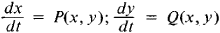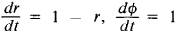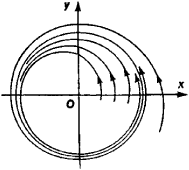# Limit Cycle

Also found in: Dictionary, Wikipedia.

## limit cycle

[′lim·ət ‚sīk·əl]
(mathematics)
For a differential equation, a closed trajectory C in the plane (corresponding to a periodic solution of the equation) where every point of C has a neighborhood so that every trajectory through it spirals toward C.
McGraw-Hill Dictionary of Scientific & Technical Terms, 6E, Copyright © 2003 by The McGraw-Hill Companies, Inc.
The following article is from The Great Soviet Encyclopedia (1979). It might be outdated or ideologically biased.

## Limit Cycle

The limit cycle of a system of second-order differential equationsis a closed trajectory in the xy-phase space which has the property that all trajectories starting in a sufficiently narrow annular neighborhood of this trajectory approach it, as t → + ∞ (stable limit cycle) and as t → –∞ (unstable limit cycle), or some approach it as t → + ∞ and the rest as t → —∞ (semistable limit cycle). For example, the system(r and ϕ are polar coordinates), whose general solution is r = 1 — (1 — r0)e-t, ϕ = ϕ0 + t (where r0 ≥ 0), has the stable limit cycle r = 1 (see Figure 1). The concept of limit cycle can be carried over to an nth-order system. From a mechanical viewpoint, a stable limit cycle corresponds to a stable periodic motion of the system. Therefore, finding limit cycles is of great importance in the theory of nonlinear oscillations.Figure 1

### REFERENCES

Pontriagin, L. S. Obyknovennye differentsial’nye urameniia, 3rd ed. Moscow, 1970.
Andronov, A. A., A. A. Vitt, and S. E. Khaikin. Teoriia kolebanii, 2nd ed. Moscow, 1959.
References in periodicals archive ?
Then there exists [[epsilon].sub.0] = [[epsilon].sub.0] ([delta]) > 0, that for all [epsilon] [member of] (0, [[epsilon].sub.0]) Equation (4.1) has an unstable limit cycle for h = (1 - [epsilon])H.
which have an invariant conic [x.sup.2] + [cy.sup.2] = 1, c [member of] R, and we prove the coexistence of large elliptic limit cycle that contains at least four small-amplitude limit cycles generated by Hopf bifurcations.
A unique unstable limit cycle appears around the equilibrium O of system (4).
then one limit cycle is bifurcated from the equilibrium [x.sup.0] at [a.sub.0].
At the same time, the nominal electric field-stretch response ([bar.E] - [lambda]) in Figure 4(b) and the nominal electric field-nominal electric displacement response ([bar.E] - [bar.D]) in Figure 4(c) show no clear limit cycle, indicating that there will be insignificant hysteresis in the viscoelastic DE for high frequency.
For [R.sub.a2] = -15.5k[ohm] a period-1 limit cycle is obtained, for [R.sub.a2] = 16.2k[ohm] a period-2 limit cycle is obtained, and chaotic attractors are obtained for [R.sub.a2] = 17k[ohm] and [R.sub.a2] = 18k[ohm].
As shown in this paper, provided that A(m[gamma]+ [delta]) < K(m[gamma]-[delta]), the phase portrait of the system of (15) is from the stable limit cycle, the orbit of which all other trajectories approach asymptotically.
In region I, two kinds of coexisting attractors, including chaotic attractor and limit cycle, are revealed.
We assumed that a hyperbolic, attractive, and stable limit cycle describes the neural oscillations [33, 34].
Caption: Figure 8: Complex limit cycle for (a) {a = 10 and d = 3} and (b) {a = 50 and d = 2}.

Site: Follow: Share:
Open / Close